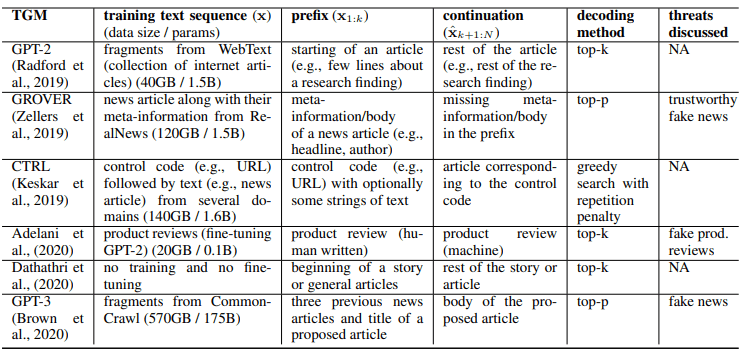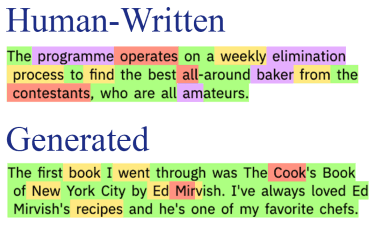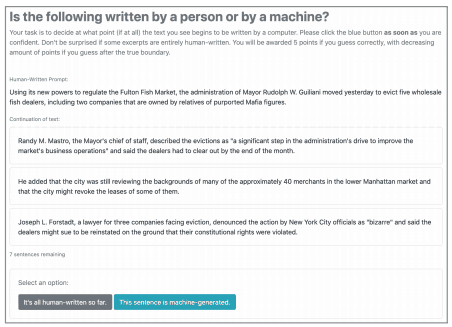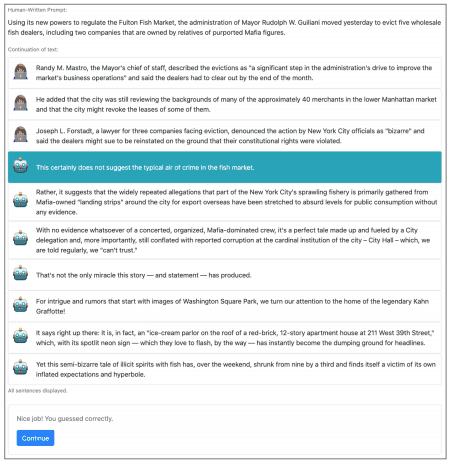# Catch up on the latest AI articles# Can You Distinguish Between Machines And Humans? An In-depth Look At The Current State Of Text Generation Models And Auto-generated Text Detection Technology!

3 main points
✔️ What is a text generation model/automatically generated text detector?
✔️ Specific examples and techniques of text generation models are explained.
✔️ Specific examples and techniques of auto-generated text detectors are explained

writt
(Submitted on 12 Nov 2020)
Comments: Accepted at The 28th International Conference on Computational Linguistics (COLING), 2020
Subjects: Computation and Language (cs.CL); Artificial Intelligence (cs.AI)## Introduction

The significant development of text generation models (TGMs) has also led to an increased risk of their misuse.

For example, the GPT-2 model was not disclosed at first because of the possibility of its use in generating fake news. In order to deter such misuse, it is important to have a technology that can distinguish between mechanically generated text and text written by humans.

This article gives a comprehensive description of the text generation model and the detection of automatically generated sentences (or texts).

1.What is the Text Generation Model (TGM)
1.1. TGM Training
1.2.Text Generation with TGM
1.2.1.Deterministic Decoding
- Greedy Search
- Beam Search
1.2.2.Probabilistic Decoding
- Unlimited sampling
- Top-k sampling
- Nuclear sampling
1.3.Social Impact of TGM

2. specific examples of text generation models (TGMs)
2.1.Main TGMs
2.2.Controllable TGM (to control the sentences generated)
2.2.1. control tokens
2.2.2. use the attribute classifier

3.Automatic Generation Sentence Detector
3.1.Classifiers trained from scratch
3.1.1.Bag-of-words classifier
3.1.2.Detecting machine configuration
3.2.Zero-shot classifier
3.2.1.Total log probability
3.2.2.Giant Language model Test Room (GLTR) tool
3.3.Fine-tuning NLM
3.3.1.GROVER detector
3.3.2.RoBERTa detector
3.4.Human-machine collaboration
3.4.1.Differences in human and machine detector
3.4.2.Supporting untrained humans
3.4.3.Real or Fake Text (RoFT) tool

4. Challenges of state-of-the-art detectors

## 1. What is Text Generation Model (TGM)

### 1.1.Learning TGM

A typical TGM is a neural language model (NLM) that has been trained to model the probability of the next token given the previous text.

For example, given the word "I", "play" as input, it predicts the probability of the word ("baseball", "soccer", etc.) following it. It can be found in $p_\theta(x_t|x_1,...,x_i,... ,x_i,... ,x_{t_1})$. Then for the token vocabulary $V$, $x_i∈V$. Let the text be denoted by $x=(x_1,... ,x_{t_1} ,x_{|x|})$, then $p_\\theta$ is usually denoted by $p_\prod(x)=\prod^{|x|}_{t=1}p_\theta(x_t|x_1,... ,x_{t-1})$ is of the form $p_{t-1}. In this case,$p_\theta$is trained to minimize the following loss function.$L(p_\theta,D)=-\l\sum^{|D|}_{j=1}\{t=1}logp_\l\theta(x^{(j)}_t|x^{(j)}|x^{(j)}_1,... ,x^{(j)}_i,... ,x^{(j)}_{t-1})$In this case,$D$represents a finite set of text from$p_*(x)$, which represents the reference distribution. ### 1.2. Text generation by TGM Given the first part of the text$x_{1:k} ~ p*$, the text generation task predicts the continuation of the given text,$p_{x}_{k+1} ~ p_\enrtheta(. |x_{1:k})$is predicted by$p_{x}_{k+1}. The resulting text $(x_1,...,x_k, and p_x_{1:k})$ is then predicted as a continuation of $(x_1,... ,x_k,\k{x}_{k+1},... ,x_x}_N)$ is targeted to increase the similarity of the sample from $p_*$.

For example, the news article generation task can be trained to generate the body of an article when given a headline. The story generation task can also generate the rest of the story from the beginning.

In this case, an approximate deterministic or stochastic decoding is used to generate a continuation of the text $\{x}_{k+1}$.

#### 1.2.1. deterministic decoding

##### Greedy search

In the greedy search, we select the token with the highest predicted probability at each time step. That is, $x_t=arg max p_\theta(x_t|x_1,... ,x_{t-1})$. In this case, the time complexity is $O((N-k)|V|)$.

##### Beam search

Beam search differs from greedy search in that it stores the top $b$ candidates with high probability. Based on these candidates, it predicts the tokens for the next time step and stores the top $b$ candidates again. This time complexity is $O((N-k)b|V|)$.

Deterministic decoding tends to generate the same words repeatedly and generic (as is acceptable in various contexts) text.

#### 1.2.2. probabilistic decoding

Probabilistic decoding involves sampling from the predicted probability distribution at each time step.

##### Unlimited sampling

Unrestricted sampling samples tokens directly from the predicted probability distribution. Although it is the simplest method, it is more likely to sample tokens with a low predicted probability (likely to be inappropriate).

##### Top-k sampling

To avoid the problems that occur with unrestricted sampling, we limit the number of tokens sampled to the top k. The appropriate value of k, in this case, depends on the context, e.g., it may need to be set higher if we are predicting nouns (large vocabulary) and lower if we are predicting prepositions (small vocabulary).

##### Nuclear (top-p) sampling

We restrict the tokens to be sampled to those with a predicted probability greater than or equal to the threshold $p$. In other words, only $p_\theta(x|x_1,... ,x_{t-1})≥p$ such that only those $x$ with $p$ are eligible for sampling. Probabilistic decoding has a tendency to produce unrealistic (such as containing large inconsistencies) statements.

### 1.3. Social impact of TGM

TGM can be exploited to generate fake news, product reviews, spam/phishing, etc. Users may not always be able to properly identify these fake news articles and reviews/comments. Therefore, in order to prevent the misuse of TGM, we need to create a model that can distinguish between human-written and auto-generated text.

## 2. Example of Text Generation Model (TGM)

### 2.1. Main TGM

The main TGMs are as follows### 2.2. Controllable TGM

Some TGMs allow you to control the topic and sentiment (positive or negative review) of an article, for example. In some cases, such information may be controlled by a given text, such as GPT-2 or GPT-3, but it may be difficult to control the generated text in detail. For this reason, the following methods have been devised to explicitly control the generated sentences.

#### 2.2.1. Control tokens

At the stage of training TGM, you enter meta-information such as the author of the news article, date of creation, and source domain as tokens. Once the training is complete, you can control the text generated by entering such meta-information.

For example, GROVER can generate news articles that are difficult to identify as fake news written by humans. CTRL also allows you to explicitly control certain attributes of the text generated by the control tokens (e.g., specifying the topic of the article to be generated). (e.g., to specify the topic of the article to be generated.)

When using control tokens, such a mechanism needs to be introduced at the time of training. This makes it difficult to use publicly available pre-training models (e.g. GPT-2) (due to high learning costs).

#### 2.2.2. Using the Attribute Classifier

In contrast, by combining TGM-generated sentences generated by TGMs such as GPT-2, for example, with some attribute classifiers (e.g., emotion classification, topic classification, etc.), the model can be adjusted to generate text containing specific attributes. In this approach, the pre-training model does not need to be trained again, and thus the generated sentences can be controlled at a low cost.

## 3. Automatic generation sentence detector

Various detectors have been devised in order to distinguish TGM-generated text from the human-written text. We will introduce some of the main research examples of such detectors.

### 3.1.Classifiers trained from scratch

Here, we'll take a look at a classifier that was trained (≈from scratch) from scratch.

#### 3.1.1.Bag-of-words classifier

A simple model using logistic regression models and tf-idf distinguishes between text in WebText and text generated by GPT-2. This is one of the simplest methods in the detector. This study tested how the detection accuracy varies for different numbers of parameters (117M, 345M, 762M, and 1542M) and sampling techniques (pure sampling, top-k sampling, and top-p sampling).

As a result, we found that the higher the number of parameters, the more difficult it was to detect, and that nuclear sampling was more difficult to detect than top-k sampling. (This may be due to the fact that top-k sampling produces more common words and the distribution of word frequencies is very different from that of humans.)

We also found that GPT-2 fine-tuning makes it more difficult to detect.

#### 3.2.2. Detecting machine configuration

Rather than classifying human-written text and auto-generated sentences as binary, this study identifies what model generated the text (e.g., decoding method, number of parameters in the model, etc.).

The results showed that the model composition was predictable (compared to the random case) with a high probability. (This task was also shown to be not as difficult as discriminating between human sentences and auto-generated sentences.) This shows that auto-generated sentences are strongly dependent on model composition.

In addition, the order of words did not play much of a role in classification, as detectors using bag-of-words (indicating the number of occurrences of a word in a sentence) performed as well as complex detectors (e.g., Transformer).

Another study showed that classical machine learning models and simple neural networks work to some extent in the following three settings.

(1) Classification of whether two articles were generated by the same TGM
(2) Classification of which TGM the article was generated from
(3) Classification of human-written and auto-generated text (target task)

However, with regard to (3), we verified the results based on several TGMs (CTRL, GPT-1, GPT-2, GROVER, XLM, XLNet, PPLM, and FAIR) and found that the raw GPT-2 text was difficult to detect.

### 3.2.Zero-shot classifier

In this section, we discuss a zero-shot classification case using pre-trained models (GPT-2, GLOVER, etc.). In this case, the model used for text generation and the model used for auto-generated sentence detection may be the same or different. In this setting, no supervised data is needed for the automatic sentence detection.

#### 3.2.1. Total log probability

As a simple example, we use TGM to evaluate the log-likelihoods. It compares the log-likelihood of a given text with the average of the log-likelihoods of the human-written and TGM-generated text, and makes predictions according to which is closer. This classifier performs poorly compared to the simple logistic regression model described above.

#### 3.2.2. Giant Language model Test Room (GLTR) tool

A technique called the GLTR tool uses differences in the distribution of text generated by GPT-2 and human-written text to perform classification. When using TGM to generate text, the tokens for the next time step are generated sequentially based on top-k sampling, nuclear sampling, etc. Now, given a text, we use some TGM to predict the probability distribution of the next token based on the first k tokens of the text. If the text is a TGM-generated sentence, we expect the distribution of predicted next tokens to be more relevant to the actual next tokens of the given text. In addition, text generated by top-k and nuclear sampling tends to have a lower frequency of unusual words.

Based on these ideas, the classification is based on the rate of occurrence of the word, the rank of the word in the predictive distribution, and entropy.

The image is shown in the following figure.In this figure, the order of the actual next tokens relative to the probability distribution of the predicted next tokens is shown in color. The upper panel shows the human text and the lower panel shows the generated text from GPT-2 large (temperature=0.7). It can be seen that many of the tokens of high rank in the distribution appear in the auto-generated text, highlighting the difference between the two texts.

### 3.3.Fine-tuning NLM

Here we are fine-tuning a pre-trained model to perform automatic sentence detection. As with zero-shot, the model used for text generation and the model used for auto-generated sentence detection may be the same or different.

#### 3.3.1. GROVER detector

In this study, we added a linear classifier on top of the GROVER model, a TGM, and fine-tuned it to perform automatic sentence detection.

We found in our experiments that the detection accuracy was better when the model used to generate the text and the model used to detect automatically generated sentences were the same. However, it is possible that the experimentally validated model just happens to show such a trend.

#### 3.3.2. RoBERTa detector

In this study, we performed fine-tuning of RoBERTa and successfully identified web pages generated by GPT-2, the largest number of parameters, with an accuracy of approximately 95%, demonstrating state-of-the-art performance.

When trained on the examples generated by core sampling, other decoding methods (top-k and unrestricted sampling) were also effective. It was also shown that detectors trained on large GPT-2 were able to adequately detect small-scale GPT-2-generated sentences. (However, in the opposite case, the detection accuracy of the large GPT-2 generated sentences is reduced.)

In addition, unlike the GROVER study described above, the RoBERTa model was more accurate when fine-tuned than when the GPT-2 model was fine-tuned. (This can be attributed to the bidirectional nature of RoBERTa.)

Another study showed state-of-the-art performance in classifying human-written and auto-generated tweets, outperforming classical machine learning models, neural networks (RNNs and CNNs), and others by a wide margin. Although RoBERTa's pre-training does not include Twitter data, it demonstrates the potential of RoBERTa to perform well on data not seen during such pre-training.

### 3.4.Human-machine collaboration

Instead of using a fully automatic detector, a combination of human and mechanical decisions could be considered. A few studies based on these attempts are described below.

#### 3.4.1.Differences in human and machine detector

The study showed that there are differences in the following capabilities for human judgment and mechanical detectors

(1) Human evaluators are better at noticing inconsistencies and errors in meaning (e.g., incoherence) in automatically generated sentences. Automatic detectors are not good at making these judgments because their ability to understand meaning is inferior to humans.
(2) If there is a word bias in the auto-generated sentences (a trend seen in top-k sampling), the auto-detectors can make the right decision, but it is difficult for human evaluation to detect these problems.

It will be important to understand these differences in capabilities if we are going to combine humans and machines for automatic sentence detection.

#### 3.4.2.Supporting untrained humans

The GLTR tool, which we discussed earlier, can help humans make decisions by visualizing the characteristics of the text. Such assistance can improve the accuracy of decisions made by humans without special training. (54% to 72%) However, the GLTR tends to be biased towards the judgment that a sentence is auto-generated, and it is difficult to determine with certainty that it is not an auto-generated sentence.

This bias leads to the need for humans and machines to "work together" as human evaluators are at risk of being drawn to mechanical decisions.

#### 3.4.2.Real or Fake Text (RoFT) tool (papers, websites )

The RoFT tool is a method for assessing the ability of humans to make decisions about auto-generated sentence detection. The human evaluator is required to detect the boundary between human-written and auto-generated sentences.

Specifically, a series of sentences, initially written by a human and then halfway through the sentence, consisting of automatically generated sentences, is given in sequence. If the user determines that the sentence has not yet been switched to automatically generated, the next sentence is given. If the user decides that it has switched to an auto-generated sentence, the user must provide a rationale for this.This procedure is repeated and evaluated to see if the evaluator can distinguish the boundaries between human-written and automatically generated sentences. The RoFT tool is actually available on this page.

As a reminder, there may be a bias, such as more human-written text being presented to users than automatically generated text.

## 4. Challenges of state-of-the-art detectors

Here we describe the challenges of state-of-the-art detectors using the RoBERTa model. This study investigates the detection task of human-written Amazon product reviews and text generated by GPT-2. We randomly investigated 100 false-positive cases where the detector failed to properly determine the false positives (i.e., when the auto-generated text was determined to have been written by a human), which included the following.

• Fluent (including rare instances of fluency that were difficult for humans to discern).
• short
• Untrue (e.g. different names of the actors in the movie reviews)
• Irrelevant content (e.g., words unrelated to music appear in music reviews)
• Contains a contradiction (A likes B but doesn't like B, etc.)
• Includes repetition (A is great, and A is great, etc.)
• Includes events that are not common sense.
• Misspellings and grammatical errors
• Incoherent content.

Thus, we have learned that there are still issues to be addressed even with the most advanced detectors, such as the inability to determine matters that can be determined to some extent by humans.

## summary

This article introduced the current state of text generation models (TGM) and automatic text detection techniques. The danger of TGM misuse is increasing with the development of text generation technologies such as GPT-2 and GPT-3. Therefore, detection technology to prevent such advanced TGM misuse will become more and more important in the future.

I hope this article will provide useful knowledge for those interested in this research area.

If you have any suggestions for improvement of the content of the article,